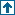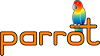parrotcode: Calculate prime numbers < 5000 Contents | Examples

# NAMEexamples/benchmarks/primes.py - Calculate prime numbers < 5000

# SYNOPSIS`    % time python examples/benchmarks/primes.py`

# DESCRIPTIONCalculates all the prime numbers up to 50000 and prints out the number of primes and the last one found.

# SEE ALSO